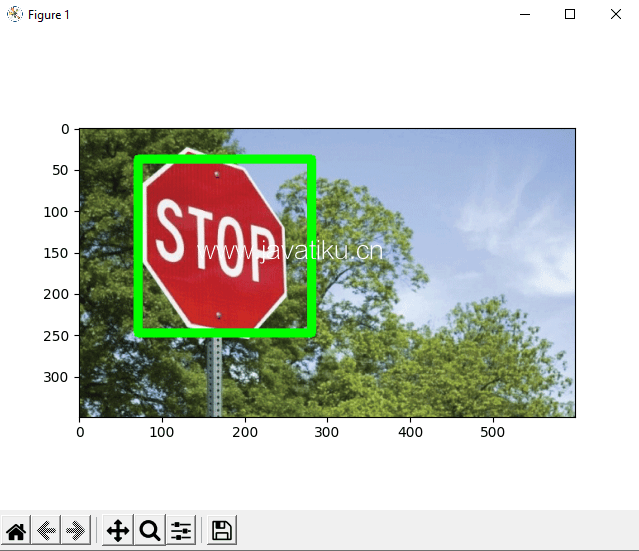# Tkinter 教程-Python OpenCV对象检测OpenCV是一个庞大且开源的图像处理、机器学习和计算机视觉库。它在实时操作中也起着重要作用。借助OpenCV库的帮助，我们可以轻松处理图像以及视频，以识别文件中的对象、人脸甚至手写文字。在本教程中，我们将只关注使用OpenCV从图像中进行对象检测。我们将学习如何使用Python程序使用OpenCV从给定图像中进行对象检测。

## 使用OpenCV进行对象检测

### Haar级联：

• 正面图像： 这些图像包含我们希望从分类器中识别出的对象。
• 负面图像： 这些图像不包含我们希望分类器检测到的任何对象，可以是其他任何东西的图像。

### 1. 安装OpenCV库：

``pip install opencv-python  ``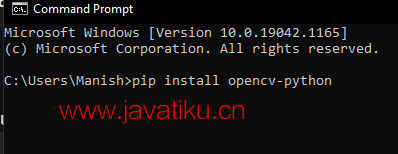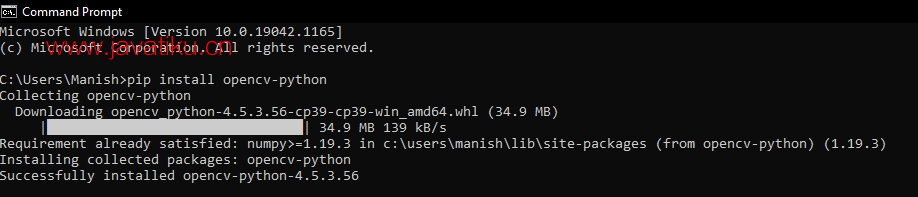### 2. 安装matplotlib库：

Matplotlib在Python程序中打开、关闭、读取等方面非常有帮助，因此在对象检测中安装此库成为重要要求。如果matplotlib库不在我们的系统中，我们必须在命令提示符终端中使用以下命令来安装它：

``pip install matplotlib ``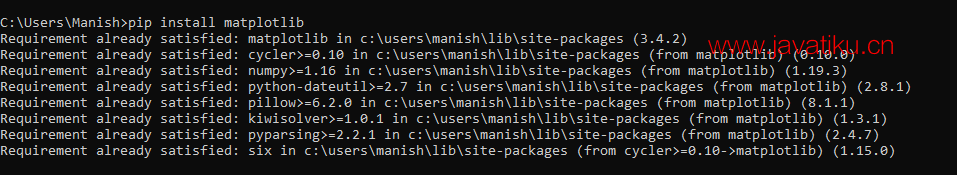### 在Python中实施对象检测：### 打开图像

``````# Import OpenCV module
import cv2
# Import pyplot from matplotlib as pltd
from matplotlib import pyplot as pltd
# Opening the image from files
# Altering properties of image with cv2
img_gray = cv2.cvtColor(imaging, cv2.COLOR_BGR2GRAY)
imaging_rgb = cv2.cvtColor(imaging, cv2.COLOR_BGR2RGB)
# Plotting image with subplot() from plt
pltd.subplot(1, 1, 1)
# Displaying image in the output
pltd.imshow(imaging_rgb)
pltd.show()  ``````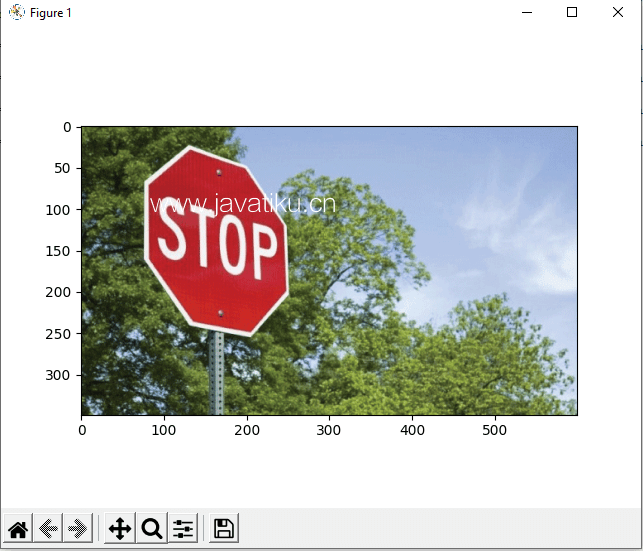### 图像中的识别或对象检测

``````found = xml_data.detectMultiScale(img_gray,
minSize = (30, 30))  ``````

``````# Import OpenCV module
import cv2
# Import pyplot from matplotlib as plt
from matplotlib import pyplot as pltd
# Opening the image from files
# Altering properties of image with cv2
imaging_gray = cv2.cvtColor(imaging, cv2.COLOR_BGR2GRAY)
imaging_rgb = cv2.cvtColor(imaging, cv2.COLOR_BGR2RGB)
# Importing Haar cascade classifier xml data
# Detecting object in the image with Haar cascade classifier
detecting = xml_data.detectMultiScale(imaging_gray,
minSize = (30, 30))
# Amount of object detected
amountDetecting = len(detecting)
# Using if condition to highlight the object detected
if amountDetecting != 0:
for (a, b, width, height) in detecting:
cv2.rectangle(imaging_rgb, (a, b), # Highlighting detected object with rectangle
(a + height, b + width),
(0, 275, 0), 9)
# Plotting image with subplot() from plt
pltd.subplot(1, 1, 1)
# Displaying image in the output
pltd.imshow(imaging_rgb)
pltd.show()  ``````